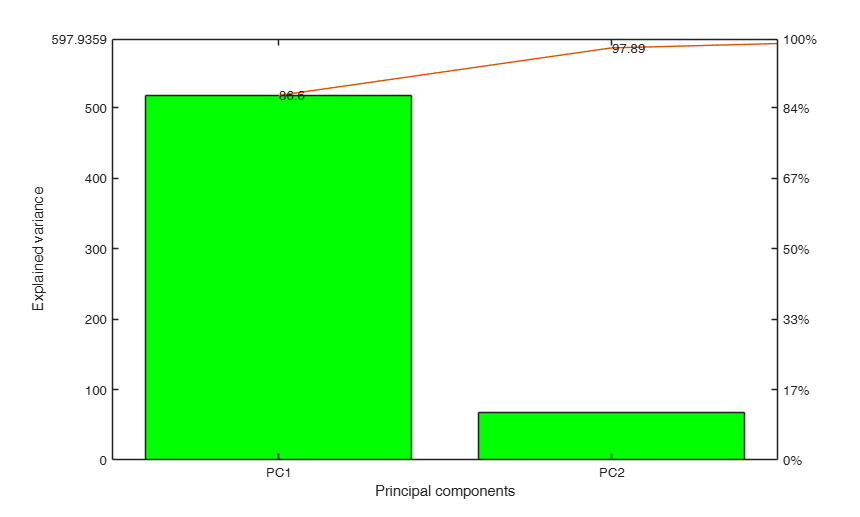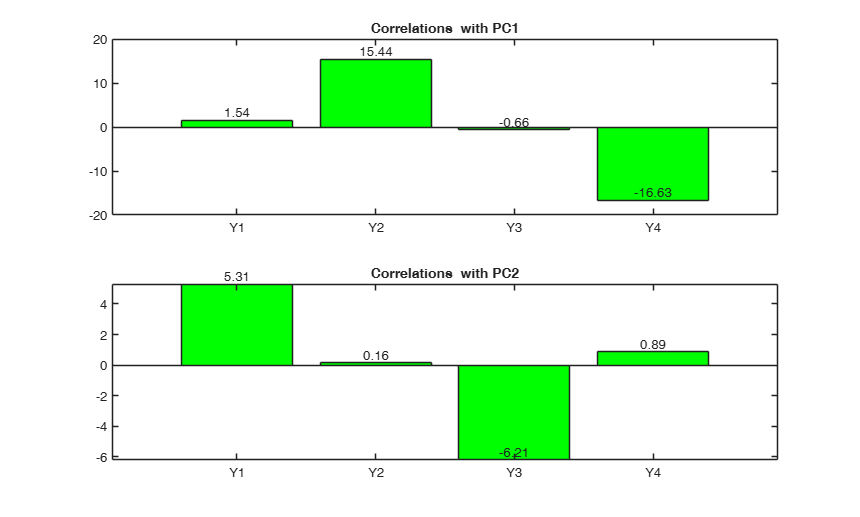pcaFS

pcaFS performs Principal Component Analysis (PCA) on raw data.

Description

The main differences with respect to MATLAB function pca are:

1) accepts an input X also as table;

2) produces in table format the percentage of the variance explained single and cumulative of the various components and the associated scree plot in order to decide about the number of components to retain.

4) provides guidelines about the automatic choice of the number of components;

5) returns the communalities for each variable with respect to the first k principal components in table format;

5) calls app biplotFS which enables to obtain an interactive biplot in which points, rowslabels or arrows can be shown or hidden. This app also gives the possibility of controlling the length of the arrows and the position of the row points through two interactive slider bars.

out =pcaFS(Y) Use of pcaFS with creditrating dataset.

out =pcaFS(Y, Name, Value) use of pcaFS on the ingredients dataset.

Examples

expand all

Use of pcaFS with creditrating dataset.

% Use all default options
out=pcaFS(creditrating(1:100,1:6))use of pcaFS on the ingredients dataset.

% Operate on the covariance matrix.
out=pcaFS(ingredients,'standardize',false,'biplot',false);
The first PC already explains more than 0.95^v variability
In what follows we still extract the first 2 PCs
Initial covariance matrix
Y1        Y2         Y3        Y4
______    _______    ______    _______

Y1     34.60      20.92    -31.05     -24.17
Y2     20.92     242.14    -13.88    -253.42
Y3    -31.05     -13.88     41.03       3.17
Y4    -24.17    -253.42      3.17     280.17

Explained variance by PCs
Eigenvalues    Explained_Variance    Explained_Variance_cum
___________    __________________    ______________________

PC1      517.80             86.60                    86.60
PC2       67.50             11.29                    97.89
PC3       12.41              2.07                    99.96
PC4        0.24              0.04                   100.00

PC1      PC2
_____    _____

Y1     0.26     0.90
Y2     0.99     0.01
Y3    -0.10    -0.97
Y4    -0.99     0.05

Communalities
PC1     PC2     PC1-PC2
____    ____    _______

Y1    0.07    0.81     0.88
Y2    0.98    0.00     0.98
Y3    0.01    0.94     0.95
Y4    0.99    0.00     0.99Input Arguments

Y — Input data. 2D array or table.

n x v data matrix; n observations and v variables. Rows of Y represent observations, and columns represent variables.

Missing values (NaN's) and infinite values (Inf's) are allowed, since observations (rows) with missing or infinite values will automatically be excluded from the computations.

Data Types: single|double

Name-Value Pair Arguments

Specify optional comma-separated pairs of Name,Value arguments. Name is the argument name and Value is the corresponding value. Name must appear inside single quotes (' '). You can specify several name and value pair arguments in any order as Name1,Value1,...,NameN,ValueN.

Example: 'standardize',false , 'plots',false , 'biplot',false , 'dispresults',false , 'NumComponents',2

standardize —standardize data.boolean.

Boolean which specifies whether to standardize the variables, that is we operate on the correlation matrix (default) or simply remove column means (in this last case we operate on the covariance matrix).

Example: 'standardize',false

Data Types: boolean

plots —plots on the screen.boolean.

If plots is true (default) it is possible to show on the screen the scree plot of the variance explained, the plot of the loadings for the first two PCs.

Example: 'plots',false

Data Types: boolean

biplot —launch app biplotFS.boolean.

If biplot is true (default) app biplotFS is automatically launched. With this app it is possible to show in a dynamic way the rows points (PC coordinates), the arrows, the row labels and control with a scrolling bar the length of the arrows and the spread of row points.

Example: 'biplot',false

Data Types: boolean

dispresults —show the results in the command window.if dispresults is true, the percentage of variance explained together with the loadings and the criteria for deciding the number of components to retain is shown in the command window.

Example: 'dispresults',false

Data Types: char

NumComponents —the number of components desired.specified as a scalar integer $k$ satisfying $0 < k \leq v$.

When specified, pcaFS returns the first $k$ columns of out.coeff and out.score. If NumComponents is not specified pcaFS returns the minimum number of components which cumulatively enable to explain a percentage of variance which is equal at least to $0.95^v$. If this threshold is exceeded already by the first PC, pcaFS still returns the first two PCs.

Example: 'NumComponents',2

Data Types: char

Output Arguments

out — description Structure

Structure which contains the following fields

Value Description
Rtable

v-by-v correlation matrix in table format.

explained

v \times 3 matrix containing respectively 1st col = eigenvalues;

2nd col = Explained Variance (in percentage) 3rd col = Cumulative Explained Variance (in percentage)

explainedT

the same as out.explained but in table format.

coeff

v-by-NumComponents matrix containing the ordered eigenvectors of the correlation (covariance matrix) in table format.

First column is referred to first eigenvector ...

Note that out.coeff'*out.coeff= I_NumComponents.

coeffT

the same as out.coeff but in table format.

v-by-NumComponents matrix containing the correlation coefficients between the original variables and the first NumComponents principal components.

score

the principal component scores. The rows of out.score correspond to observations, columns to components. The covariance matrix of out.score is $\Lambda$ (the diagonal matrix containing the eigenvalues of the correlation (covariance matrix).

scoreT

the same as outscore but in table format.

communalities

matrix with v-by-2*NumComponents-1 columns.

The first NumComponents columns contain the communalities (variance extracted) by the the first NumComponents principal components. Column NumComponents+1 contains the communalities extracted by the first two principal components. Column NumComponents+2 contains the communalities extracted by the first three principal components...

communalitiesT

the same as out.communalities but in table format.